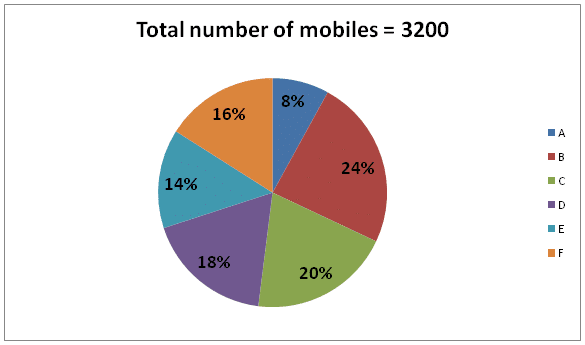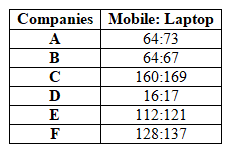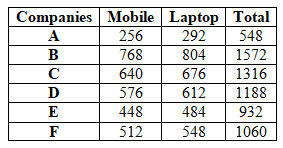# RRB PO Prelims Quantitative Aptitude Questions 2021 (Day-04)

Dear Aspirants, Our IBPS Guide team is providing new series of Quantitative Aptitude Questions for RRB PO Prelims 2021 so the aspirants can practice it on a daily basis. These questions are framed by our skilled experts after understanding your needs thoroughly. Aspirants can practice these new series questions daily to familiarize with the exact exam pattern and make your preparation effective.

Start Quiz

Missing number series

Directions (1-3): What value should come in the place of (?) in the following number series?

1) 7, 20, 37, 56, 79, ?

A.111

B.108

C.118

D.105

E.115

2) 26, 54, 181, 527, ?, 2596

A.1080

B.1120

C.1180

D.1260

E.1220

3) 24, 36, 72, 180, 540, ?

A.1890

B.1880

C.1900

D.1920

E.1840

Pie chart

Directions (04-08): Study the following information carefully and answer the questions given below.

There are six different companies – A, B, C, D, E and F and they manufactured the number of mobile and laptops.

Total number of manufactured products of the company = number of mobile manufactured + number of laptops manufactured.

The given pie chart shows the number of mobiles manufactured in six different companies.The given table shows the ratio of the number of mobile and laptop manufactured in six different companies.4) What is the difference between the total number of products manufactured in A and C together and the total number of products manufactured in D and F together?

A.360

B.396

C.384

D.392

E.376

5) Number of mobile manufactured in A and B together is approximately what percent of the total number of products manufactured in E?

A.110%

B.115%

C.117%

D.119%

E.108%

6) What is the ratio of the number of mobile manufactured in F to the number of laptop manufactured in D?

A.14:19

B.64:71

C.18:25

D.19:24

E.None of these

7) What is the average number of laptop manufactured in D and E together?

A.548

B.550

C.552

D.554

E.556

8) If the average number of products manufactured in B and G together is 1376 and number of mobile manufactured in G is 960, then what is the number of laptop manufactured in G?

A.180

B.190

C.200

D.220

E.240

9) What is the average number of products manufactured in all the companies together (approximately)?

A.1100

B.1103

C.1110

D.1105

E.1112

Probability

10) A bag contains 3 Apple, 4 Orange and 5 Banana. If two fruits are drawn at random, then what is the probability that both are Orange?

A.1/11

B.2/11

C.3/11

D.4/11

E.None of these

7+ 13 =20

20 + 17 = 37

37 + 19 = 56

56 + 23 = 79

79 + 29 = 108

26 +33+ 1= 54

54 +53+ 2 = 181

181 +73 + 3 = 527

527 +93 + 4 = 1260

1260 +113 + 5 = 2596

24 * 1.5 = 36

36 * 2 = 72

72 * 2.5 = 180

180 * 3 = 540

540 * 3.5 = 1890Required difference = (1188 + 1060) – (548 + 1316)

= 384

Required percentage = (256 + 768)/932 * 100 = 110%

Required ratio = 512: 612

= 128:153

Required average = (612 + 484)/2

= 548

Total number of products manufactured in B and G = 1376 * 2 = 2752

Number of products manufactured in G = 2752 – 1572 = 1180

Number of laptop in G = 1180 – 960 = 220

Required average = (548 + 1572 + 1316 + 1188 + 932 + 1060)/6 = 1102.67 =1103